# GRE Calculator: 4 Top Tips for How to Use It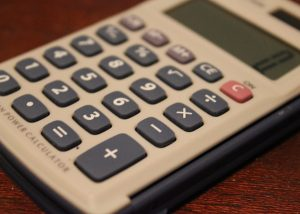Can you use a calculator on the GRE general test? Absolutely, but you cannot bring your own calculator to use on test day. If you take the computerized test, you’ll be able to use the simple, on-screen GRE calculator for the Quantitative Reasoning sections; if you take the paper test, a similar calculator will be provided to you.

Make sure you’re using the right kind of calculator in your GRE studying! Learn more about the calculator that’s provided to you on the GRE general test with this guide. I’ll cover exactly what all the buttons do and how to use the GRE calculator to improve your score.

feature image credit: Calculator/used under CC BY 2.0/Cropped from original.

## The GRE Calculator: What Can It Do?

The on-screen calculator you’ll see when taking the GRE looks like this: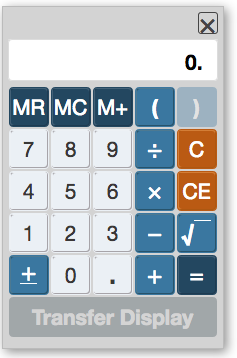You can choose to show or hide the on-screen calculator throughout the Quantitative Reasoning sections – you don’t have to have it on your screen for every question, potentially distracting you and taking up valuable screen real estate.

The GRE calculator allows you to add, subtract, divide, multiply, parenthesize, take the square root of, add a decimal to, or change the sign (positive/negative) of numbers. You can also store and recall answers for future use using the MR, MC, and M+ keys, same as with a normal four-function calculator.

One final function of the GRE calculator allows you to transfer the answer from the display exactly to the answer box. This feature can be helpful, but it also comes with some problems (more on that later).

Quick side note: we've created the world's leading online GRE prep program that adapts to you and your strengths and weaknesses. Not sure what to study? Confused by how to improve your score? We give you minute by minute guide.

You don't NEED a prep program to get a great GRE score. But we believe PrepScholar is the best GRE prep program available right now, especially if you find it hard to organize your study schedule and don't know what to study.

### GRE Calculator Display

The calculator display is 8 digits long, including decimals.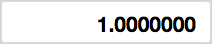It automatically demarcates numbers greater than 100 in standard American form (comma after every three digits, right to left):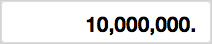If you try to calculate a number that is greater than eight digits (e.g. 10,000,000 multiplied by 10,000,000), you’ll get an error message: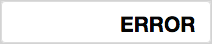On the other hand, if a number has more than eight decimal places, like 1/10,000,000/9, the calculator display will show “0”: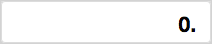Even though it displays 0, however, the calculator will still “know” the real answer, such that if you then try to multiply by, say, 10,000,000, the calculator will show you the correct answer, so if you multiply by 10,000,000, it will give you: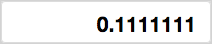## GRE Calculator Buttons: Numbers & Functions

In the image below, I’ve labeled each of the non-numerical functions of the GRE calculator. I’ll go through all of the functions below.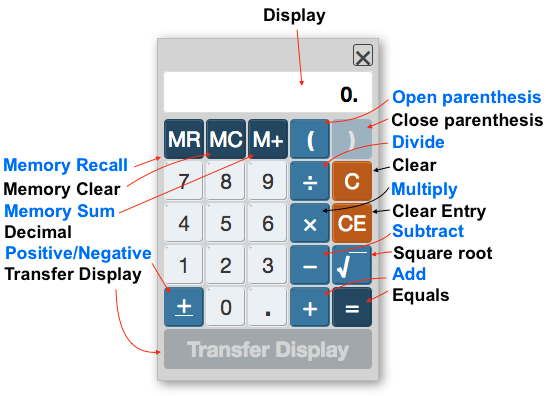Note: A blue outline appears around the calculator and a white outline around the transfer display button when the calculator is selected, like so: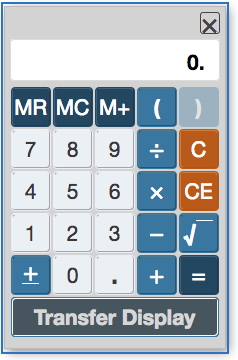If your calculator has this blue outline, then it will recognize any commands you type using your computer keyboard.

### Numbers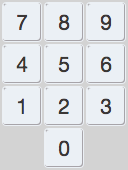The calculator has digits 0-9, arranged like a phone keypad. You can enter using numbers by using the computer keyboard or by clicking on numbers individually with the cursor.

### C (Clear Input)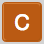Clear all input. This doesn’t clear the memory if you’ve stored something using M+, but it does clear any other functions and numbers you’ve entered into the calculator and sets the default screen back to 0.

### CE (Clear Entry)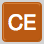The Clear Entry button clears the last number you put in, but it doesn’t get rid of the whole equation. For example, let’s say you wanted to divide 16184.88 by 4, but accidentally put in “5” instead of 4, CE would get rid of the 5 and allow you to put in 4 without having to retype in “16184 ÷”.

This can be time-saving if you have a lengthy equation like (16184.88-3216)/4. In general, though, you can get by without using CE and just going with plain old “C” instead and reentering the calculation.

Clicking “C” or “CE” on the calculator, however, will not clear your answer from the answer area on the test. You must go into the numeric entry box and separately remove your answer. For example, let’s say you accidentally transferred “42” to the numeric entry box: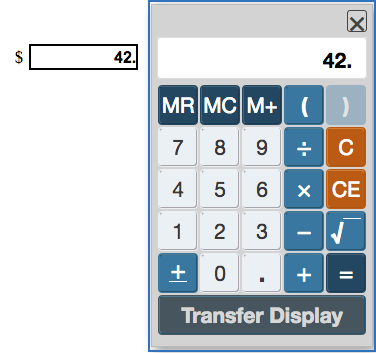If you press “C” or “CE,” the calculator display will clear…but the answer entry will not.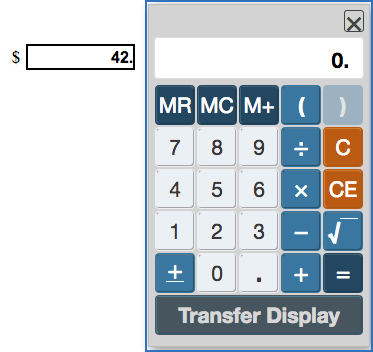### . (Decimal)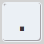Keyboard shortcut: .

Use this button to delineate decimals, like 3.14 or 2.7. The GRE uses the American system of decimals so that they’re written using a period, not a comma. For example, 200 + 15/100 would be written 200.15 (not 200,15).

### +/- (Positive/Negative)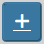Use this button to toggle back and forth between positive or negative for a number.

Want to improve your GRE score by 7 points? We have the industry's leading GRE prep program. Built by world-class instructors with 99th percentile GRE scores, the program learns your strengths and weaknesses through machine learning data science, then customizes your prep program to you so you get the most effective prep possible.

Try our 5-day full access trial for free: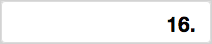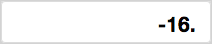Unlike with many computer calculators, you can’t use the keyboard shortcut “-” to make a number negative — you must use this button.

### √ (Square Root)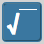Use this button to take the square root of a number. You must first enter in the number you want to take the square root of, then click then “root” sign.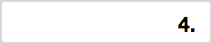If you try to take the square root of a negative number, you’ll get an error message.### ÷ (Divide)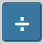Keyboard shortcut: /

Use this function for division of one number by the other.

### x (Multiply)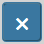Keyboard shortcut: *

Use this function for multiplication of two numbers

### – (Subtract)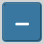Keyboard shortcut: –

Use this function for subtraction of one number by the other.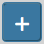Keyboard shortcut: +

Use this function to add two numbers together

### () (Parentheses)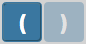Keyboard shortcuts: ( )

Parentheses are useful for manipulating order of operations. Like most cellphone calculators these days, the GRE calculator follows PEMDAS (parentheses, exponents, multiplication, division, addition, subtraction). This means that the part of the equation in parentheses is the part that gets processed first, followed by any division/multiplication, then any addition/subtraction.

For instance, look at the following equation:

(4+6)/5 = 2

Without parentheses, the calculator automatically starts with division, so if you leave them out you’ll get

4 + 6/5 = 5.2

Want to improve your GRE score by 7+ points?

Check out our best-in-class online GRE prep program. We guarantee your money back if you don't improve your GRE score by 7 points or more.

PrepScholar GRE is entirely online, and it customizes your prep program to your strengths and weaknesses. We also feature 2,000 practice questions, official practice tests, 150 hours of interactive lessons, and 1-on-1 scoring and feedback on your AWA essays.

Check out our 5-day free trial now:

because the calculator divides 6 by 5 before adding 4. To add 4 to 6 first, you need to use parentheses. And yes, with numbers this small (4+6) you probably wouldn’t be using the calculator anyway, but this example can be applied to more complex numbers.

Important note: There are no nested parentheses on the GRE calculator (probably because you can’t actually see the parentheses on the display, so it would be hard to keep track of the nesting). This means that you cannot, for instance, do something like

((16/2)/(43-59))-64

on the GRE calculator. Instead, you’d need to solve the individual parts of the equation first until it’s reduced down to one set of parentheses, like so:

(-8/16)-64 = -64.5

### = (Equals)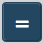Keyboard shortcut: =

You can also use “return” or “enter” key on the keyboard as a shortcut. Use this button to get a result of a function (addition, subtraction, multiplication, division, and so on). The equals sign is also useful for getting around the calculator’s order of operations.

Take the above example:

((16/2)/(43-59))-64

Instead of solving 16/2, 43-59, and then plugging it all in, you can just use the equals sign to answer it one step at a time.

16/28(43-59)-0.564-63.5

### M+ (Memory Sum)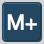To store an answer for later use in calculations, hit “M+”. An M will appear to the left of the display to show that an answer’s been stored.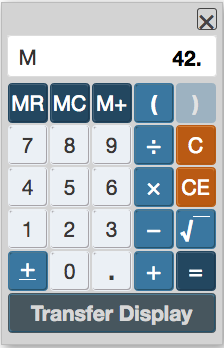You can then clear the answer using “C” – an M will remain on the left side of the display to indicate there’s something stored in the memory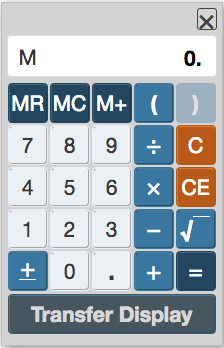### MR (Memory Recall)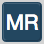To recall a stored answer, hit MR. You can do this to begin a calculation (e.g. MR – 8) or at the end of a calculation (e.g. 86/MR).

### MC (Memory Clear)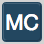To clear the stored answer, hit MC. You’ll know it was successful when the “M” disappears from the lefthand side of the display.

These three memory functions (M+, MR, and MC) are useful for problems that involve complex equations, since they allow you store

My advice about the memory functions: If you’re able to become comfortable with them (or you already are comfortable with them), great! Use them to your advantage. If you don’t want to invest the time into learning how to use the buttons and instead prefer to use some combination of parentheses, equals, and writing down numbers on scratch paper…that’s also perfectly acceptable, and way better than getting nervous and misusing the functions on the GRE.

Bottom line: only use M+, MR, and MC if they make the GRE easier for you.

### Transfer Display

For most types of questions on the Quantitative Reasoning section (all Quantitative Comparison and Multiple Choice questions), the “Transfer Display” button will appear grayed out, as below: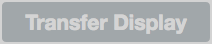When you do come across Numeric Entry questions where the transfer display button can be used (and have used the calculator), the transfer display button turns darker grey: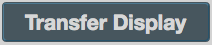You can then click “transfer display” to transfer answer from calculator to the answer area on the test.

While it can be useful, the “Transfer Display” button has a couple of quirks it’s important to know about ahead of time:

1. Transfer display does not work with questions with fractional answers, even if they’re Numeric Entry boxes – you have to manually enter in the numbers to the top and the bottom of the fraction.
2. Transfer display will transfer information from the calculator even if the answer has a different requirement (i.e. even if the answer must be a positive number or only contain a certain number of decimal digits). See below for an example: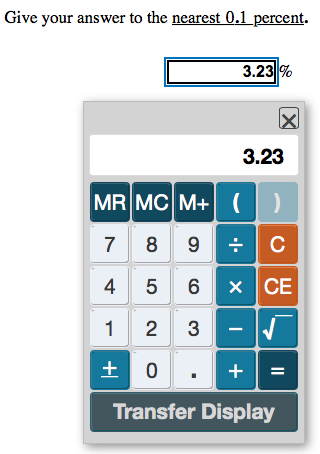That doesn’t look rounded to one decimal place to me, GRE calculator!

### What’s Not on the GRE Calculator?

The GRE on-screen calculator has some functions missing that you might normally expect to find on your average scientific calculator.

• No exponents or squaring or cubing. If you want to find out, for instance, what 32 cubed is, you have to manually multiply out 32 times 32 times 32 on the GRE calculator.
• No constants like π or e.
• No logarithmic (ln, log) or trigonometric (sine, cosine, tangent) functions.
• No nested parentheses (see above discussion of the GRE calculator’s parentheses function).

## Paper-Based GRE Calculator

What about if you’re taking the paper-based test: can you use a calculator on the GRE? Yes, you can, though there are different rules and regulations governing the use of calculators.

On paper-based GRE general tests, you’ll be given a calculator to use on the Quantitative Reasoning section. You can’t use your own calculator, so don’t bring one to the test!

The paper-based GRE calculator is very similar to the computerized one: it’s a basic four-function calculator, with a square root button and memory functions. The main difference is in how it handles order of operations — instead of being based on PEMDAS, it’s instead based on order of entry, left to right.

Take the example from earlier of 4 + 6/5. On the physical calculator ETS gives you, the answer (if you don’t use parentheses) will be 2, because the calculator will automatically read 4 + 6/5 as (4+6)/5 = 2.

The best way to practice for this is to buy your own four-function calculator (i.e. one that looks like the GRE calculator) to use in practice. Remember, though, you won’t be able to take an outside calculator with you into the test, so don’t get too attached to a specific calculator.

## GRE Calculator Tips and Tricks

We’ve covered what you can do with the GRE calculator above, but how can you use a calculator on the GRE effectively? I’ve collected some of the best tips for using this tool below.

### #1: Solve Problems Without the Calculator When You Can

Don’t use the GRE calculator for simple math that would be quicker to solve without a calculator. Not only is it simpler to solve things like (4*8) or (2400/3) without going through the calculator, but it also cuts down on entry errors (i.e. accidentally typing in 2400/4) that could affect your answer.

### #2: Use the Computer Keyboard When Possible

Use the keyboard of the computer, rather than clicking each number/function, to save time. You should be able to use numbers on the keyboard enter in numbers to the calculator (although you may not be able to use the num pad).

Similarly, you may be able to use other common shortcuts like *, -, /, +, =, and the return/enter key instead of having to click the functions on the calculator individually (and go back and forth between keyboard and mouse). The one limitation is that you can’t use the backspace, delete, or C on the keyboard to clear the calculator display.

### #3: Be Careful of Order of Operations

Keep order of operations straight by using parentheses and the equal sign. If all else fails, you can solve for one part of an equation at a time, write what that answer is on scratch paper, solve for the next part, write that down, and so on. But using parentheses and the “equals” function speeds up the process tremendously.

### #4: Pay Attention to Error Messages

If you find you’re reaching the limits of the calculator’s capabilities (for example, too many digits for the screen), you’re probably making an error in your approach to the question. No question will ask you to go beyond the limits of the calculator’s abilities. For example, if a question has exponents in it, you’ll be expected to use your knowledge of the rules of dividing, adding, multiplying, raising to a power, and factoring exponents, rather than brute force approaches of multiplying everything out.

## Bottom Line: What You Need to Know About the GRE Calculator

1. Can I use a calculator on the GRE? Yes, on the Quantitative Reasoning (i.e. math) section.
2. Can you take a calculator to the GRE? No. You’ll use an on-screen calculator on the computer GRE and be given a four-function calculator to use with the paper GRE.
3. Try out the GRE calculator online by getting PowerPrep.
4. Remember that, like your phone calculator, the GRE calculator follows order of operations – use parentheses accordingly.
5. Most problems on the Quantitative Reasoning section are faster to solve without the calculator, so don’t rely too heavily on the calculator.

## What’s Next?

You can have a calculator on the GRE, but what about scrap paper? We have the scoop about scratch paper on the GRE.

What can you expect from the GRE besides a calculator? Discover more about the GRE test format and what’s on the GRE.

Thinking about taking the GRE, but not sure when’s the best time? Find out how long you need to study for the GRE and read our complete guide to choosing the best GRE test date for you.

## Ready to improve your GRE score by 7 points?

We've written a eBook about the top 5 strategies you must be using to have a shot at improving your GRE score. Download it for free now:## Author: Laura Staffaroni

Laura graduated magna cum laude from Wellesley College with a BA in Music and Psychology, and earned a Master's degree in Composition from the Longy School of Music of Bard College. She scored 99 percentile scores on the SAT and GRE and loves advising students on how to excel and fulfill their college and grad school dreams.